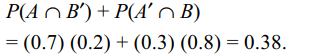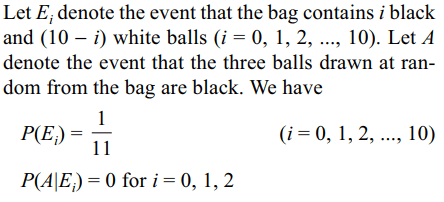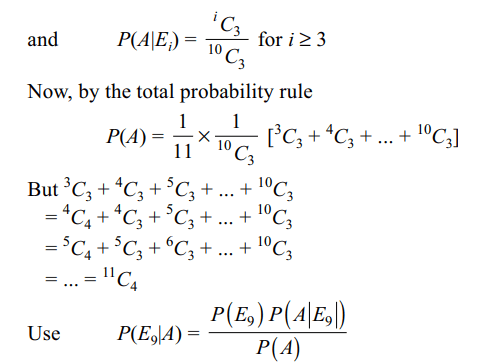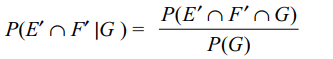## Probability Questions and Answers Part-11

1. A speaks the truth in 70 percent cases and B in 80 percent cases. The probability that they will contradict each other in describing a single event is
a) 0.36
b) 0.38
c) 0.4
d) 0.42

Explanation:2. 2n boys are randomly divided into two subgroups containing n boys each. The probability that the two tallest boys are in different groups is
a) $\frac{n}{2n-1}$
b) $\frac{n-1}{2n-1}$
c) $\frac{2n-1}{4n^{2}}$
d) $\frac{n-1}{2n^{2}}$

Explanation:3.The numbers 1, 2, 3, .... , n are arranged in random order. The probability that the digits 1, 2, .... , k (k < n) appears as neighbours in that order is
a) $\frac{1}{n!}$
b) $\frac{k!}{n!}$
c) $\frac{\left(n-k\right)!}{n!}$
d) $\frac{\left(n-k+1\right)!}{n!}$

Explanation: 1, 2, 3, .... k appear together in that order in (n - k + 1)! ways

4. Two boys and three girls stand in a queue. The probability the number of boys ahead of every girl is at least one more than the number of girls ahead of her
a) 1/2
b) 1/3
c) 2/3
d) 0

Explanation: Impossible event

5. A bag contains some white and some black balls, all combinations of balls being equally likely. The total number of balls in the bag is 10. If three balls are drawn at random without replacement and all of them are found to be black, the probability that the bag contains 1 white and 9 black balls is
a) 14/55
b) 12/55
c) 2/11
d) 8/55

Explanation:6. Let E, F, G be pairwise independent events with P (G) > 0 and $P\left(E\cap F\cap G\right)=0$     . Then $P\left(E'\cap F' \mid G\right)$    equals
a) $P\left(E'\right)+P\left(F'\right)$
b) $P\left(E'\right)-P\left(F'\right)$
c) $P\left(E'\right)-P\left(F\right)$
d) $P\left(E\right)-P\left(F'\right)$

Explanation:7. A and B are two events. The probability that at most one of A, B occurs is
a) $1-P\left(A\cap B\right)$
b) $P\left(A'\right)+P\left(B'\right)-P\left(A'\cap B'\right)$
c) $P\left(A'\right)+P\left(B'\right)+P\left(A\cup B\right)-1$
d) All of the Above

Explanation: All of the Above

8. The probability of the simultaneous occurrence of two events A and B is p. If the probability that exactly one of A, B occurs is q, then
a) $P\left(A'\right)+P\left( B'\right)=2-2p-q$
b) $P\left(A\cap B \mid A\cup B\right)=\frac{p}{p+q}$
c) $P\left(A'\cap B' \right)=1-p-q$
d) All of the Above

Explanation: All of the Above

9. Suppose that P(A) = 3/5 and P(B) = 2 3. Then
a) $P\left(A\cup B \right)\geq 2/3$
b) $4/15 \leq P\left(A\cap B \right)\leq 3/5$
c) $2/5 \leq P\left(A\mid B \right)\leq 9/10$
d) All of the Above